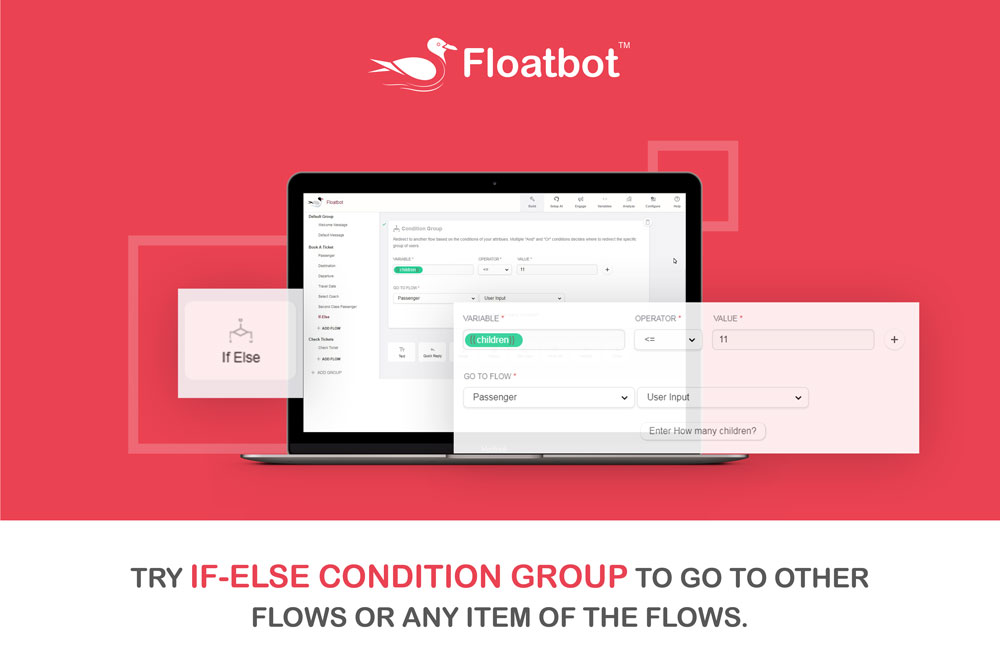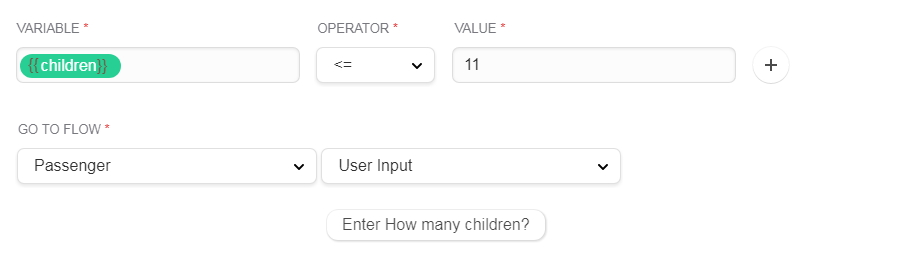Feb 21, 2018

# Floatbot is Live with its If-Else Feature

One more feature is live for you guys!!

The If-Else feature controls the flow of response based on the user’s attribute. With this feature, you can redirect the user to a specific flow or item of the flow which is predetermined by you.

To Specify Condition

• Select variable from the predefined variables.
• Select the operator
• Enter the value for comparison with the variable.
• Select the Go to flow from predefined flows.
• Select the item from the Go to flow, where the flow will be redirected if the condition gets true.

If you don’t want to specify condition, then just select Go to flow, and the item inside the flow, (by default 1st item of the flow will be selected).

Acceptable Operators are:

• == If the value equals variable
• !=  If the value not equals variable
• <  If the value is less than variable
• >  If the value is greater than variable
• <= If the value is less than or equal to value
• >=  If the value is greater than or equal to value
• Is Set  If the variable value is set
• Is Not Set  If the variable value is not set
• Like If the variable is like the value

Let us see an example of this feature.

This is an example of online railway ticket portal. And here we are adding a flow using this feature asking if the age of the children is 11.

The image below shows condition groups of an online ticket booking chatbot. After completing the input details. The variable is inserted with {{children}} and value of 11. Below screenshot shows the use of the variable and value in this bot.Here if the value of the children will be less than or equal to 11 then it will go to “Passenger” flow to the User Input with the message “Enter How many children?” that is shown below.

Try the feature by yourself Here and give us your feedback !!